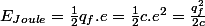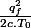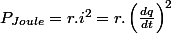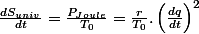# Entropy change in an RC circuit

Hello,I would like some help for a problem

## Homework Statement

Initially:At t=0 [/B]the cylindrical capacitor of capacitance $c=\frac{\epsilon s}{d}$ (d the distance between the 2 electrodes and s their surface; $\epsilon = \epsilon(T)$ is the dielectric permittivity) is discharged and we close the circuit and charge isothermally the cylindrical capacitor until it has a $c*v$ charge.
The current is $i=\frac{dq}{dt}$ and the voltage is v(t), the electric charge of the capacitor is $q(t)=c*v(t)$.
We have an RC circuit receiving work $W$ from a generator of voltage $v$ and energy $Q_{exchanged}$ from a thermal reservoir (thermostat) of $T_0$ Kelvin,the circuit has its entropy change$\Delta S_{in}$ during the charging.
We have $C_q$ the heat capacity for a constant charge q and $\lambda= \frac{qT \epsilon'}{\epsilon c}$ another coefficient in the equation :
$TdS_{in}= C_q dT + \lambda dq$ (1)

What is the entropy of the universe $\Delta S_{univ}$ equal to ?
Does $C_q$ depend on $q$ ?

## Homework Equations

$\Delta S_{thermostat}=\frac{Q_{exchanged}}{T_0}$

## The Attempt at a Solution

If we integrate $C_q$ over T ,I think q does appear in the formula for $C_q$ but it's not a variable I think.To find $\Delta S_{in}$ we divide the equation (1) by T then integrate $\frac{\lambda}{T}$ over q from 0 to q=cv.
$\Delta S_{univ}=\Delta S_{thermostat}+\Delta S_{in}$
I would like to know how to express $\Delta S_{thermostat}$ and it's sign. Thank you.

What physical physical picture you are trying to work with? That is, what phenomenon are you interested in? It seems you have the capacitor bathed in a temperature reservoir. Why?

What physical physical picture you are trying to work with? That is, what phenomenon are you interested in? It seems you have the capacitor bathed in a temperature reservoir. Why?

I want to know how to express the entropy change , I think the entropy change of the universe is 0 but I'm not sure what the equation is.The RC circuit is getting heat from the reservoir and work from the generator,I'm guessing it's to make it a reversible process.

I am not sure, but I suspect you are confused. Ordinarily one does not consider heat when analyzing an RC circuit, and the capacitance of a capacitor does not vary much with temperature. I am not so sure that the net change in entropy of the universe is zero; For irreversible processes, entropy increases.

Generally the flow of current through a circuit with resistance is not a reversible process. The resistors give off heat but never absorb heat.

•yamata1
rude man
Homework Helper
Gold Member
This is pretty advanced thermodynamics. I can only give you some ideas:
Since the capacitor is being charged isothermally, dT = 0 and you are left with dS = $\lambda dq$/T = (ε'/εc)q dq. You didn't say what ε' and c are. But your eq. (1) is of course a form of the Maxwell "2nd T dS equation" so there must exist a coefficient representing the change of polarization with T ( related by the state equation). So maybe ε' = dε/dT and ΔSdielectric = (ε'/εc) q dq from q=0 to q = Cv.
Then the total change in entropy of the universe is just ΔSdielectric - ΔSthermostat.

Sorry, not very definite; hope others will do better.

I think I have an explanation :

The generator gives the system {resitance,capacitor} the electric energy :The capacitor charges itself by en absorbing half ( the work ##W=\int vdq##). The difference represents the lost energy through the Joule effect :This energy is received through heat by the heat reservoir at fixed temperature ,which increases the entropy by :Accouting for the resistance, we have :
$$\Delta S_{univ}=\frac{\varepsilon'}{2\varepsilon.c}\cdot q_{f}^{2}-\frac{\varepsilon'}{2\varepsilon.c}\cdot q_{f}^{2}+\frac{q_{f}^{2}}{2c.T_{0}}=\frac{q_{f}^{2}}{2c.T_{0}}$$

there is a creation of entropy caused by the Joulet effect which is an irreversible phenomenon as Gene Naden wrote
I am not sure, but I suspect you are confused. Ordinarily one does not consider heat when analyzing an RC circuit, and the capacitance of a capacitor does not vary much with temperature. I am not so sure that the net change in entropy of the universe is zero; For irreversible processes, entropy increases.

Generally the flow of current through a circuit with resistance is not a reversible process. The resistors give off heat but never absorb heat.
.
The power received by the heat reservoir is the power produced by the Joule effect :